# Convert the following LEX program into Lexical Analyzer. AUXILIARY DEFINITIONS − − − TRANSLATION RULES a{ } abb{ } a*b+

Compiler DesignProgramming LanguagesComputer Programming

#### Web Design for Beginners: Build Websites in HTML & CSS 2022

68 Lectures 8 hours

#### HTML5 & CSS3 Site Design

61 Lectures 6 hours

## Solution

• Convert the pattern into NFA’s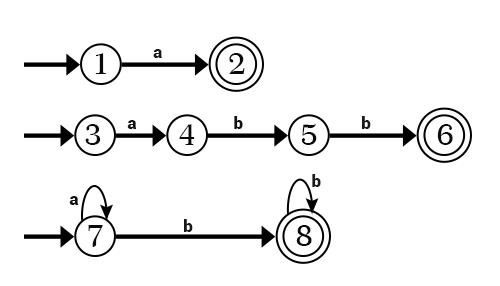• Make a Combined NFA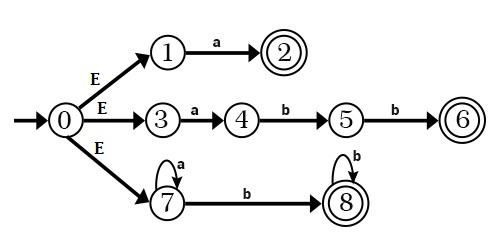• Convert NFA to DFA

A = ε − closure (0) = {0, 1, 3, 7}

The transition on symbols a, b from state A

For State A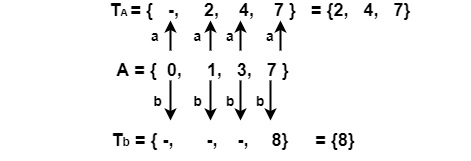ε − closure (Ta)                              ε − closure (Tb)

= ε − closure ({2, 4, 7})                  = ε − closure ({8})

= {2, 4, 7} = B                                = {8} = C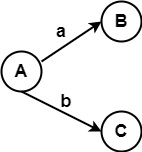For State B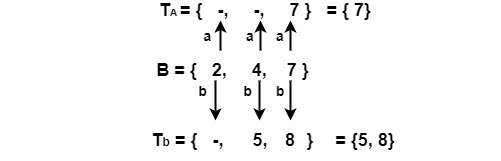ε − closure (7) = {7} = D                ε − closure ({5, 8}) = {5, 8} = E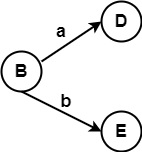For State C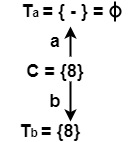ε − closure (ф) = ф |                     ε − closure (8) = {8} = C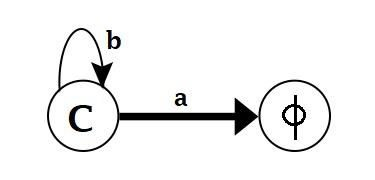For State D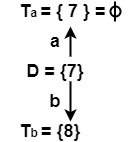ε − closure (7) = {7} = D | ε − closure (8) = {8} = C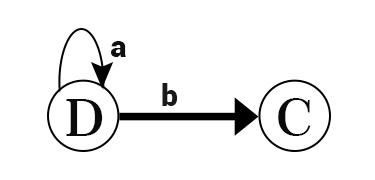For State E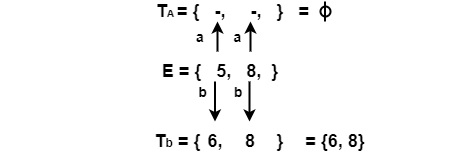ε − closure (ф) = ф | ε − closure {(6, 8)} = {6, 8} = F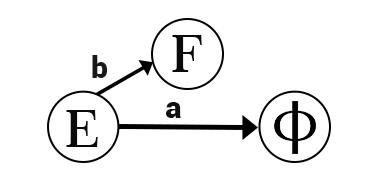For State F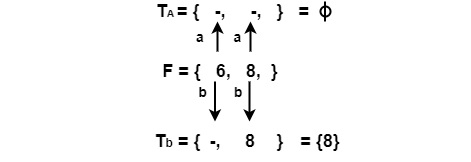ε − closure (ф) = ф | ε − closure (8) = {8} = C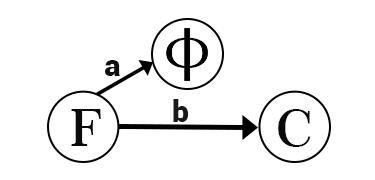Combining all transition Diagrams, we get a complete DFA. Since states 2, 6, 8 are final states in NFA.

States in NFA having their states, i.e., 247, 8, 58, 68 are final states.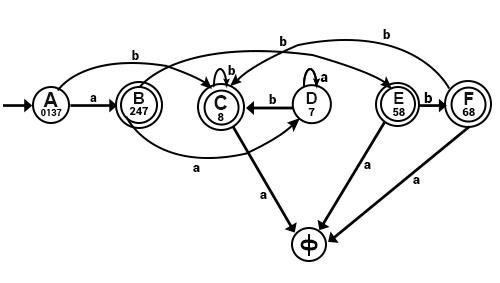StateAbToken Recognize
01372478none
247758a
88a*b+
778none
5868a*b+
688abb
none

Tokens Recognized

0137 → No state in {0, 1, 3, 7} is Final State. Therefore, no token will be recognized by this state.

247 → State 2 in this state is the final state. State 2 accepts a combined NFA. Therefore, 247 will accept a.

8 → 8 is the Final State in combined NFA. It accepts a*b+ in the combined NFA.

7 → 7 is not the final state & therefore it accepts nothing.

58 →8 is the final state, but 5 is a non-final state. State 8 accepts a∗b+in combined NFA. Therefore 58 will accept a*b+.

68 → Both states 6 & 8 are final states. But 6 accepts abb, and 8 accepts a*b+ in combined NFA. But abb comes before a*b+ in the Translation rules. Therefore state 68 will accept token abb.